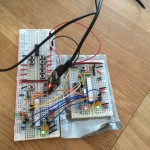# HIL testing in Arduino

I am trying out Hardware-in-the loop simulation on a new Arduino project for a client. Test driven development is unfortunately not very big in the Arduino community (yet) so I decided to implement something by myself. The setup is simple:

1. One Arduino is running the application software.
2. Another Arduino connects to inputs and outputs on the first Arduino. This Arduino will include all the test code.A test case could for example be, when the user presses a button a LED should light up. The second Arduino will output a signal to the button input on the first Arduino, and then check if the LED pin output is high. Example code is shown below.

```void loop() {
// Simulate that user presses button
digitalWrite(BUTTON_PIN, 1);

// check that the LED lights up
assert(LED_PIN, 1);

delay(500)

// check that some actuator starts running
assert(ACTUATOR_PIN, 1);

// Simulate that user releases button
digitalWrite(BUTTON_PIN, 0);

// Led and actuator should turn off
assert(LED_PIN, 1);
assert(ACTUATOR_PIN, 1);

// stop execution
while (1) {}
}

bool assert(uint8_t pin, bool expectedState) {
if (state != expectedState) {
Serial.print("## FAILURE: pin ");
Serial.print(pin);
Serial.print(" == ");
Serial.print(state);
Serial.print(" != ");
Serial.print(expectedState);
Serial.println();
return false;
}
else {
Serial.print("## OK: pin ");
Serial.print(pin);
Serial.print(" == ");
Serial.print(state);
Serial.println();
return true;
}

}```

## Why the hassle?

It might seem unnecessary, (and it is for simple problems), but it does increase code quality and decreases the risk of bugs being introduced when writing new features to the code.

## Making it better

I would like to write my test code in Python on a laptop and control an Arduino (via Firmata for example). Then I would have proper tools for testing and generating test reports. For now the Arduino solution is sufficient though.

Kategori: Arduino, Blog
###### 2 Comments »HIL testing in Arduino
1.Danee skriver:

Hello Sebastian Nilsson,

•sebnil skriver: